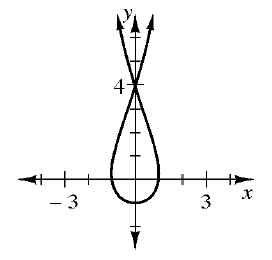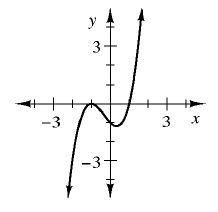### Home > GB8I > Chapter 1 Unit 1 > Lesson INT1: 1.2.2 > Problem1-47

1-47.

Which of the relationships below are functions? If a relationship is not a function, give a reason to support your conclusion.

1.There is more than one output value for some inputs; therefore, the relation is not a function.

1. input $\boldsymbol {(x)}$

output $\boldsymbol {(y)}$

$–3$

$19$

$5$

$19$

$19$

$0$

$0$

$–3$

It is a function. Every input had only one output even if it is the same output such as $19$.

1.  input $\boldsymbol {(x)}$ output $\boldsymbol {(y)}$ $7$ $–2$ $0$ $7$ $4$ $10$ $0$ $10$ $3$ $0$

There is more than one output value for $x = 7$. Is this relation a function?

1.It is a function.